# Electric force on the charge kept at the centre of a metallic shell

Pushoam
Homework Statement:
A metallic spherical shell contains a charge Q on it. A point charge q is placed at the centre of the shell and another charge ##q_1## is placed outside. All the three charges are positive the force on the charge at the centre is ..... and the force on the central charge due to the shell is.....
Relevant Equations:
Electric field inside a conducting shell due to the shell or outside charge is zero.
Hence the electric force on the charge in both cases is zero. Is this correct?

Homework Helper
Gold Member
2022 Award
Electric field inside a conducting shell due to the shell or outside charge is zero.
That is not quite right.

Pushoam
That is not quite right.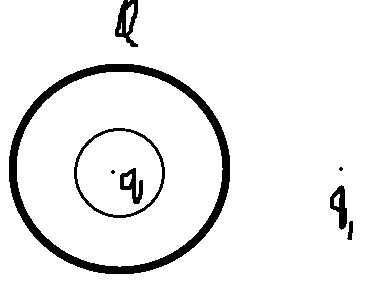For calculating electric field inside the shell due to shell and outside charge ## q_1##, let's consider that there is no charge at the center.
Due to ## q_1## the charge Q is not uniformly distributed on the metallic sphere. Hence, the electric field due to this inside the sphere is nonzero.
Let's take a spherical Gaussian surface. Then, the charge enclosed by this surface is 0. Applying Gauss's law,
$$\oint (\vec E_{shell} +\vec E_{outside~ charge}) \cdot d\vec A = 0$$ .....(1)
## \vec E_{shell} \neq 0##
## \vec E_{outside~ charge} \neq 0. ##

Electric field inside (in the meat of) a conductor is 0. Hence, ## \vec E_{shell} +\vec E_{outside~ charge}= 0## in the meat of the shell.
Electric field at the surface of a conductor is perpendicular to its surface as any tangential component gets cancelled by the movement of chargedparticles. Hence, electric field at the inner surface of the shell is radially inward (and the presence of charge q at center doesn't affect this direction). Hence, from (1), ## \vec E_{shell} +\vec E_{outside~ charge}= 0 ## inside the shell.

Thus the force on the central charge is 0. At centre, the electric field due to ## q_1 ## is towards left. Hence, the electric field and force due to the shell is towards right.

Last edited:
Homework Helper
Gold Member
Note that $$\oint (\vec E_{shell} +\vec E_{outside~ charge}) \cdot d\vec A = 0$$does not imply that ##\vec E_{shell} \neq 0## and ##\vec E_{outside~ charge} \neq 0.## It's like saying that (5 -3)+(6-8) = 0 implies that (5-3)= 0 and (6-8) = 0.

Also, you did not specify the Gaussian surface that you chose. I suggest a closed surface that is entirely inside the metallic shell.

Pushoam
Note that $$\oint (\vec E_{shell} +\vec E_{outside~ charge}) \cdot d\vec A = 0$$does not imply that ##\vec E_{shell} \neq 0## and ##\vec E_{outside~ charge} \neq 0.## It's like saying that (5 -3)+(6-8) = 0 implies that (5-3)= 0 and (6-8) = 0.
Due to ## q_1## the charge Q is not uniformly distributed on the metallic sphere. Hence, the electric field due to this inside the sphere is nonzero.
Hence,
$$\oint (\vec E_{shell} +\vec E_{outside~ charge}) \cdot d\vec A = 0$$ .....(1)
## \vec E_{shell} \neq 0##
## \vec E_{outside~ charge} \neq 0. ##
Also, you did not specify the Gaussian surface that you chose. I suggest a closed surface that is entirely inside the metallic shell.
The Gaussian surface is concentric spherical and drawn in the picture.

I edited my last post #3 after your post #4.

Last edited:
Homework Helper
Gold Member
The Gaussian surface is concentric spherical and drawn in the picture.
The picture also shows charge ##q## enclosed by the Gaussian surface. Is that the case or is that not the case? If there is no charge inside the shell and you think that the electric field inside the shell is not zero, then draw an electric field line that you think might be appropriate inside the shell. Be mindful of the fact that electric field lines start at positive charges and end at negative charges, i.e. they point from a region of high electric potential to a region of low electric potential.

Homework Helper
View attachment 321690

Due to ## q_1## the charge Q is not uniformly distributed on the metallic sphere. Hence, the electric field due to this inside the sphere is nonzero.
What do you mean by "this"? q1 or Q? If you mean Q, this is not right.
This would imply that the field inside a metallic body is zero only if the body is spherical symmetric. If you put charge on a potato-shaped conductor the charge density is not uniform. Would you say that the field inside this conductor is not zero?

Pushoam
The picture also shows charge ##q## enclosed by the Gaussian surface. Is that the case or is that not the case? If there is no charge inside the shell and you think that the electric field inside the shell is not zero, then draw an electric field line that you think might be appropriate inside the shell. Be mindful of the fact that electric field lines start at positive charges and end at negative charges, i.e. they point from a region of high electric potential to a region of low electric potential.
The charge q is there at the center. For calculating electric field due to charge Q on the shell and ## q_1## outside inside the shell, I have taken no charge at the center.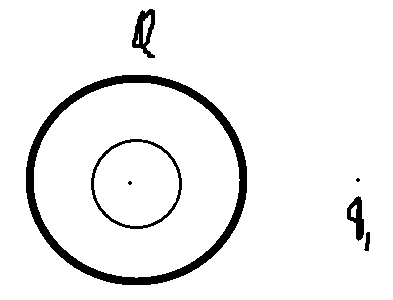The net electric field inside the shell is 0, but electric field due to the shell's charge Q and outside charge ## q1## separately are non-zero.

That is what I meant in #3.

Pushoam
What do you mean by "this"? q1 or Q? If you mean Q, this is not right.
This would imply that the field inside a metallic body is zero only if the body is spherical symmetric. If you put charge on a potato-shaped conductor the charge density is not uniform. Would you say that the field inside this conductor is not zero?
I meant Q.
I did not mean that
the field inside a metallic body is zero only if the body is spherical symmetric.
Here, by inside, I do not mean the meat part of the conductor. Here, by inside of shell I meant the volume bounded by the shell.

The shell is a symmetric body. So, if Q is uniformly distributed on the shell, then electric field due to Q inside the shell is 0.

Here, due to ##q_1## outside, the charge Q is not uniformly distributed on the shell. Hence, electric field due to Q inside the shell is nonzero.

Homework Helper
The field inside the metal is zero no matter how the charge is distributed on the surface. And the same hold true for the hole inside, if there is no charge inside. If, for a given distribution Q on the surface, the field is zero everywhere inside the metal, this will not change if, maintaining the Q as it is, a hole is cut inside the metal.

Homework Helper
Gold Member
The shell is a symmetric body. So, if Q is uniformly distributed on the shell, then electric field due to Q inside the shell is 0.
It is true that the shell is a symmetric body.
It is not true that ##Q## is uniformly distributed on the shell if there is point charge ##q_1## outside the shell.
My two questions to you are: If there is no charge inside the shell and if charge ##Q## is not uniformly distributed over the shell, is the electric field inside the shell zero? Why or why not?

Homework Helper
Gold Member
2022 Award
It is true that the shell is a symmetric body.
It is not true that ##Q## is uniformly distributed on the shell if there is point charge ##q_1## outside the shell.
As noted by @Pushoam in the paragraph following the one you quoted.

Homework Helper
Gold Member
As noted by @Pushoam in the paragraph following the one you quoted.
Yes, but it's the justification of the "Hence" that begins that paragraph that I am really after by asking @Pushoam to answer the two questions in tandem hoping that he might reconsider the conclusion.

Pushoam
Hence, electric field due to Q inside the shell is nonzero.
If there is no charge inside the shell and if charge Q is not uniformly distributed over the shell, is the electric field inside the shell zero? Why or why not?
The net electric field ( ## \vec E_{shell} +\vec E_{outside~ charge}##) inside the shell is zero. This comes from Gauss's law (as there is no charge enclosed by the Gaussian surface).

Homework Helper
Gold Member
No, this does not come from Gauss's law. No charge enclosed does not guarantee that the field inside is zero. Look at the picture below that shows a positive charge and spherical shell with no charge enclosed.

If the shell material is dielectric, the electric field inside the shell is not zero.
If the shell material is conducting, the field inside the shell is zero.

Gauss's law is satisfied in both cases in that the surface integral of the normal component of the electric field is zero in agreement with zero enclosed charge. So Gauss's law doesn't cut it.

There is another reason why the field inside the conductor is zero that has nothing to do with Gauss's law but a lot to do with the difference between conductors and dielectrics. Can you figure it out?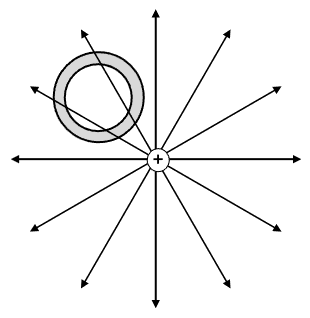Pushoam
There is another reason why the field inside the conductor is zero that has nothing to do with Gauss's law but a lot to do with the difference between conductors and dielectrics. Can you figure it out?
Applying Gauss's law, $$\oint (\vec E_{shell} +\vec E_{outside~ charge}) \cdot d\vec A = 0$$
Electric field at the surface of a conductor is perpendicular to its surface as any tangential component gets cancelled by the movement of charged particles. Hence, electric field at the inner surface of the shell is radially inward (and the presence of charge q at center doesn't affect this direction).
A conductor has free charge particles which distribute themselves such that

1) net charge and electric field within the meat of the conductor is 0 and
the tangential component of electric field on the surface gets 0 as if these being non-zero lead to the movement of electron until they become 0.

2) the component of electric field perpendicular to the surface is nonzero as charged particles can't move in this direction.

3) the conducting surface is equipotential as different potentials at different part of the surface leads to the movement of charge particles such that the potentials become equal.

Hence, the electric field at the inner surface of conductor is radially inward as the charges are positive.

Since, there is no charged particle within shell, the direction of the electric field inside the shell is unaffected and hence radially inward.
Now, $$\vec A = A \hat r$$ $$\vec E_{shell} +\vec E_{outside~ charge} =( E_{shell} +E_{outside~ charge})(-\hat r)$$ $$\oint (\vec E_{shell} +\vec E_{outside~ charge}) \cdot d\vec A = 0$$ $$\oint -( E_{shell} + E_{outside~ charge}) d A = 0 ~~~ .......(1)$$

If I assume that ## E_{shell} + E_{outside~ charge} ## is constant over the Gaussian surface, then it has to be 0 for (1) to hold true.

Last edited:
Pushoam
If the shell material is dielectric, the electric field inside the shell is not zero.
In case of dielectric, taking the effect of polarisation as negligible, and initial Q charge distribution to be uniform,
1) the Q charge distribution stays unaffected due to the presence of outside charge ##q_1##.
2) Hence, the ## E_{shell} = 0## due to the symmetry of the system can be derived using Gauss's law.
3) ## \vec E_{net} = \vec E_{outside ~ charge} = \frac {q_1}{4 \pi \epsilon_0 r^2 } \hat r## where r is distance from charge ## q_1## and ## \hat r## is the radially outward direction centered at ##q_1##.

Homework Helper
Gold Member
I understand and I agree with items 1, 2 and 3 in post #16. I don't understand this
Hence, the electric field at the inner surface of conductor is radially inward as the charges are positive.
Then you say
Since, there is no charged particle within shell, the direction of the electric field inside the shell is unaffected and hence radially inward.
I have some difficulty picturing this and I don't want to guess what picture you have in your mind. Can you post a sketch of what this electric field might look like inside the conductor? I am talking about the conducting shell with no charge inside but a charge outside as shown in post #15. Thanks.

Homework Helper
Gold Member
2022 Award
I've lost track of how this discussion relates to the problem in post #1 (which makes no mention of dielectrics).

Pushoam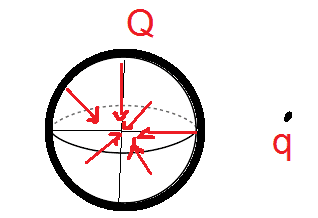I don't understand this

According to 1) and 2) in post#16, the tangential component of the net electric field at the conducting surface is 0 and it has only non-zero perpendicular component of net electric field. Hence, the net electric field has to be perpendicular to the shell's surface i.e radial. Since, Q and ##q_1## are positive, the electric field originates from them towards the shell. Hence, it is radially inward.

The electric field inside the shell is not due to Q and ## q_1##.
The charges distributes themselves in such a way that there is no electric field inside the meat of the shell. To cancel the electric field due to ##q_1## and positive charge on the outer surface, some positive charged particles get distributed on the inner surface.

The electric field is due to the positive charges present on the inner surface of the shell. The inner surface is equipotential. Hence, the electric field is perpendicular to the surface and away from the surface i.e. towards radially inward direction.

Since, I do not know the magnitude of the net electric field, hence I draw them as non-constant. The electric field near the surface is considered to be higher as it is closed to the positive charge there and according to Coulomb's law, electric field is inversely prorportional to ##r^2##.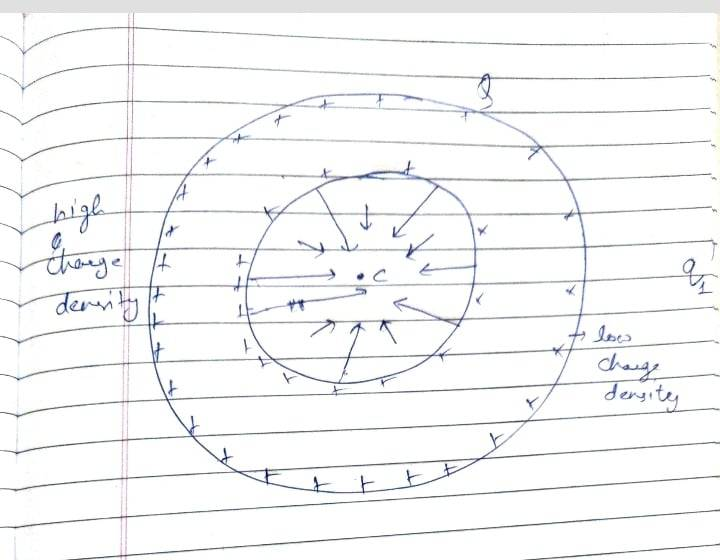Last edited:
Pushoam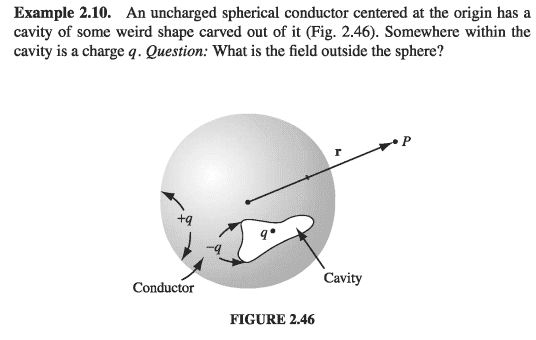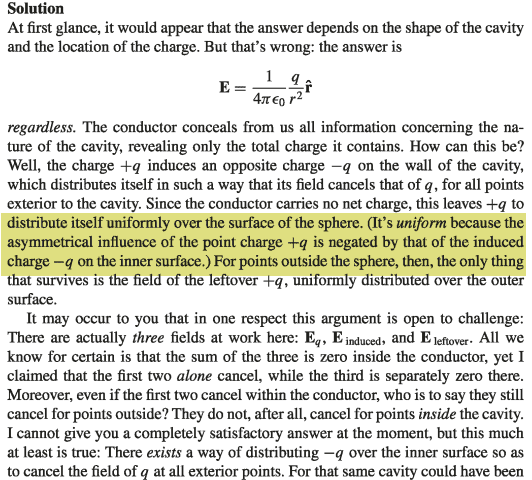The above images have been taken from Griffith's Introduction to Electrodynamics.
Considering the highlighted part, the non-uniform charge distribution occurs only at the outer surface. The charge distribution at the inner surface is uniform. This distribution rersults into net electric field being 0 in the meat of the shell as well as inside the shell.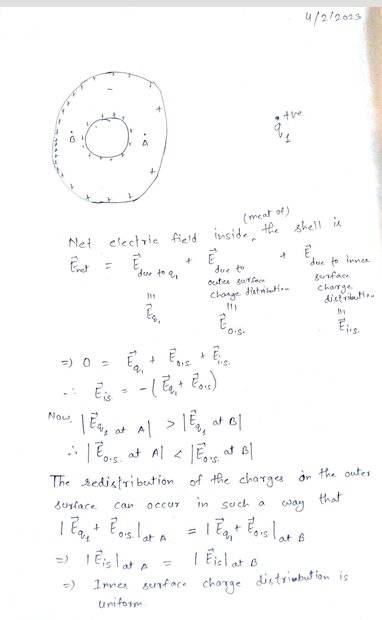Pushoam
My two questions to you are: If there is no charge inside the shell and if charge ##Q## is not uniformly distributed over the shell, is the electric field inside the shell zero? Why or why not?
Under the effect of ##q_1## the redistribution of charge Q takes such that it is non-uniform on the outer surface and uniform on the inner surface. This distribution results into net electric field being 0 in the meat of the shell as well as inside the shell.

Pushoam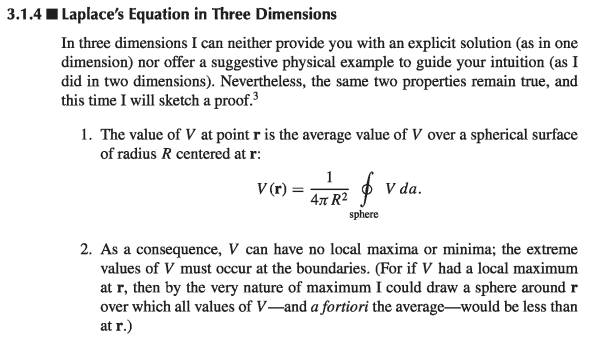The above image is taken from Griffith's Introduction to Electrodynamics.

Taking ##\vec r## as the center of the shell and the inner surface of shell as the surface for integration, potential at center equals potential at the surface from 1st property.

From second property, there can be no local maxima or minima, implying V being constant in the volume covered by the inner surface. Hence, in this region ## \vec E_{net} = -\nabla V = 0##.

Homework Helper
Gold Member
I've lost track of how this discussion relates to the problem in post #1 (which makes no mention of dielectrics).
Here is ball of thread to guide you through the labyrinth of posts herein. Initially, OP seems to think that if a charge is outside a charged conducting shell with a cavity, where there is no charge (post #16), there is a radially inward electric field inside the cavity:
Since, there is no charged particle within shell, the direction of the electric field inside the shell is unaffected and hence radially inward.
Then there is stuff where Gauss's law was introduced in a way that I don't quite understand to conclude with the statement
If I assume that ## E_{shell} + E_{outside~ charge} ## is constant over the Gaussian surface, then it has to be 0 for (1) to hold true.
The identity of "it" is that "has to be 0" is not clear to me. Probably the electric field inside the cavity but that would directly contradict OP's earlier statement that the electric field inside the cavity is radially inward. That is why I asked the OP to sketch and post the field inside the cavity which was done in post #20. OP seems not to understand that this sketch violates Gauss's law which should be the first order of business because OP seems not to understand what Gauss's law is saying.

I introduced the dielectric shell with cavity in the presence of an external charge as a counterexample to convince the OP that Gauss's law is not the way to go if one wishes to show that the electric field in the cavity of the conductor is zero. The Gauss's law argument (whatever that is) that OP uses to show that field inside the conductor cavity is zero (where it is actually zero or so I thought OP thought) should be applicable to show that the field inside the dielectric cavity where we know that the electric field is not zero. How can the same Gauss's law argument produce different results depending on the shell material? I tried to get the OP to see that Gauss's law is not the basis for why the electric field inside the conductor cavity is zero. I now see that OP needs to understand what Gauss's says before proceeding any further.

I hope this helps you find your way through this morass of posts and textbook references and zero in what OP understands and what OP does not understand. My next step is to show how the picture in #20 violates Gauss's law.

•Pushoam
Pushoam
That is why I asked the OP to sketch and post the field inside the cavity which was done in post #20. OP seems not to understand that this sketch violates Gauss's law which should be the first order of business because OP seems not to understand what Gauss's law is saying.
I am sorry.
That field which I drew in post#20 is wrong as that indicates presence of negative charge in the cavity.

•kuruman
Homework Helper
Gold Member
I am sorry.
That field which I drew in post#20 is wrong as that indicates presence of negative charge in the cavity.
I'm glad you realized that because it saved me some work. So is there an electric field inside the conducting shell when there charge ##Q## on the shell, charge ##q_1## outside the shell and no charge inside the shell? If "yes", please post a revised drawing of the cavity with the field inside that satisfies Gauss's law. If "no", explain why not.

•Pushoam
Pushoam
Electric field at the surface of a conductor is perpendicular to its surface as any tangential component gets cancelled by the movement of chargedparticles. Hence, electric field at the inner surface of the shell is radially inward (and the presence of charge q at center doesn't affect this direction). Hence, from (1), ## \vec E_{shell} +\vec E_{outside~ charge}= 0 ## inside the shell.
The above conclusion is wrong.
The electric field inside the shell and on its inner surface can neither be radially inward nor in any other direction. Hence, it has to be 0.

Pushoam
I introduced the dielectric shell with cavity in the presence of an external charge as a counterexample to convince the OP that Gauss's law is not the way to go if one wishes to show that the electric field in the cavity of the conductor is zero. The Gauss's law argument (whatever that is) that OP uses to show that field inside the conductor cavity is zero (where it is actually zero or so I thought OP thought) should be applicable to show that the field inside the dielectric cavity where we know that the electric field is not zero. How can the same Gauss's law argument produce different results depending on the shell material? I tried to get the OP to see that Gauss's law is not the basis for why the electric field inside the conductor cavity is zero.
I understood this. Thanks for it.
Gauss's law doesn't lead to the conclusion that electric field inside the conducting shell is 0. It's the redistribution of charged particles which leads to 0 electric field inside the conducting shell.

•SammyS
Homework Helper
Gold Member
It's the redistribution of charged particles which leads to 0 electric field inside the conducting shell.
You need to be more specific here. Suppose that the charges on the inside surface of the cavity redistribute themselves due to the external charges ##Q## and ##q_1## so that there are field lines inside the cavity but not inside the meat of the conductor (see schematic below.) One could argue that the field inside the meat of the conductor is zero as it should. Also, this arrangement satisfies Gauss's law. If I draw a Gaussian surface inside the meat of the conductor, the net flux through the surface is zero and so is the net enclosed charge. The same is true if I draw a Gaussian surface inside the cavity because all the field lines that enter the surface leave it and there is no enclosed charge. So what is the argument that says that the picture as shown below cannot be the case? Try to be as specific as you can.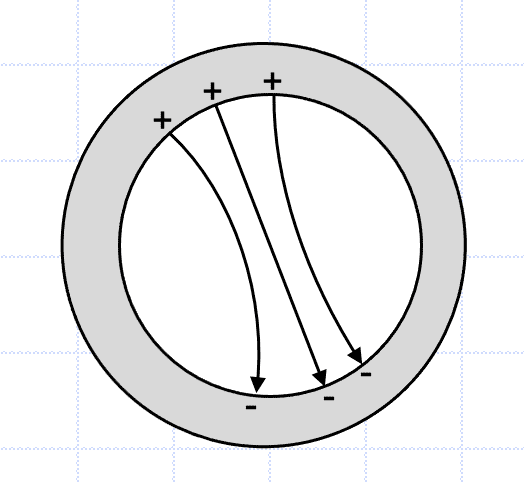•Pushoam
Pushoam
One could argue that the field inside the meat of the conductor is zero as it should. Also, this arrangement satisfies Gauss's law. If I draw a Gaussian surface inside the meat of the conductor, the net flux through the surface is zero and so is the net enclosed charge. The same is true if I draw a Gaussian surface inside the cavity because all the field lines that enter the surface leave it and there is no enclosed charge. So what is the argument that says that the picture as shown below cannot be the case? Try to be as specific as you can.

View attachment 321748
The above charge distribution doesn't guarantee 0 electric field inside the meat of the shell.
The inner surface of a conducting shell has to be equipotential.
In the given diagram, potential near the negative charge is not equal to the potential near the positive charge. Besides this charge distribution also creates a component of electric field along the inner surface. The charges will move on the inner surface to make it equipotential and 0 electric field.

Just because the diagram satisfies the Gauss's law doesn't mean that electric field inside the meat is 0.
To cancel the field due to ##q_1## in the meat of the shell, a part of charge Q distributes on the upper surface and rest of Q on the inner surface. So, the negative charge can not stay there on its own. It gets neutralised due to the presence of other positive charge.

Last edited:
Pushoam
So what is the argument that says that the picture as shown below cannot be the case? Try to be as specific as you can.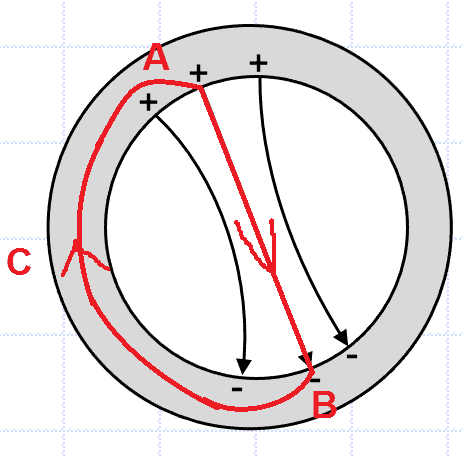Using ## \oint \vec E \cdot d \vec l =0 ## for the closed loop ABCA shown in the figure, where the part AB inside the cavity is along the direction of electric field, to calculate the magnitude of ## \vec E## inside the cavity, $$\oint \vec E \cdot d \vec l =0$$$$\int_A^B \vec E \cdot d \vec l + {\int_B^A }_{through~ C} \vec E \cdot d \vec l = 0$$$$\int_A^B \vec E \cdot d \vec l = -{\int_B^A }_{~ through~ C} \vec E \cdot d \vec l$$

Now, ## {\int_B^A }_{through~ C} \vec E \cdot d \vec l = 0## as electric field inside the meat of conducting shell is 0.
Hence, $$\int_A^B \vec E \cdot d \vec l = -{\int_B^A }_{through~ C} \vec E \cdot d \vec l = 0 ... (1)$$ As the part AB inside the cavity is along the direction of electric field, $$\int_A^B \vec E \cdot d \vec l =\int_A^B E dl =0$$$$\Rightarrow E = 0 \Rightarrow \vec E = \vec 0$$

Hence, the above calculation and precisely, ## \oint \vec E \cdot d \vec l =0## says that there can be no electric field inside the shell.

Last edited:
•kuruman
••